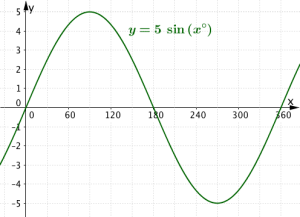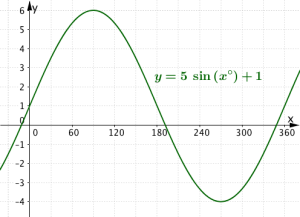# Sine Function Properties: Range and Period

The graph of the functionhas a minimum and a maximum value of. The minimum value is; the maximum value is. The period of this curve is 360.

## Vertical Stretch,; Vertical Translation:Now when this graph is stretched and translated vertically, the minimum and maximum values change.

The amount of vertical stretch will tell you how far on theaxis it is from ‘the bottom of the trough to the top of the crest’. For example when we multiplyby 5, we get the curvewhich has a minimumvalue at -5; maximum at 5 – the distance on theaxis between top and bottom is 10.Now let’s translate this curve 1 unit upwards – we get the curve. Now the min is -4; the max is +6 – because the whole curve has been moved up 1 unit.## Horizontal Stretchand the period of the curve

Now a sinusoidal function of the formthat hashas period 360 – it has not undergone a horizontal stretch. When, it undergoes a horizontal stretch of scale factor(a compression scale factor). Consider the graph. The parameter, therefore the period is.You may find it helpful to remember the formula:orIn conclusion, the graphhas range [-4, 6] and has period 180.